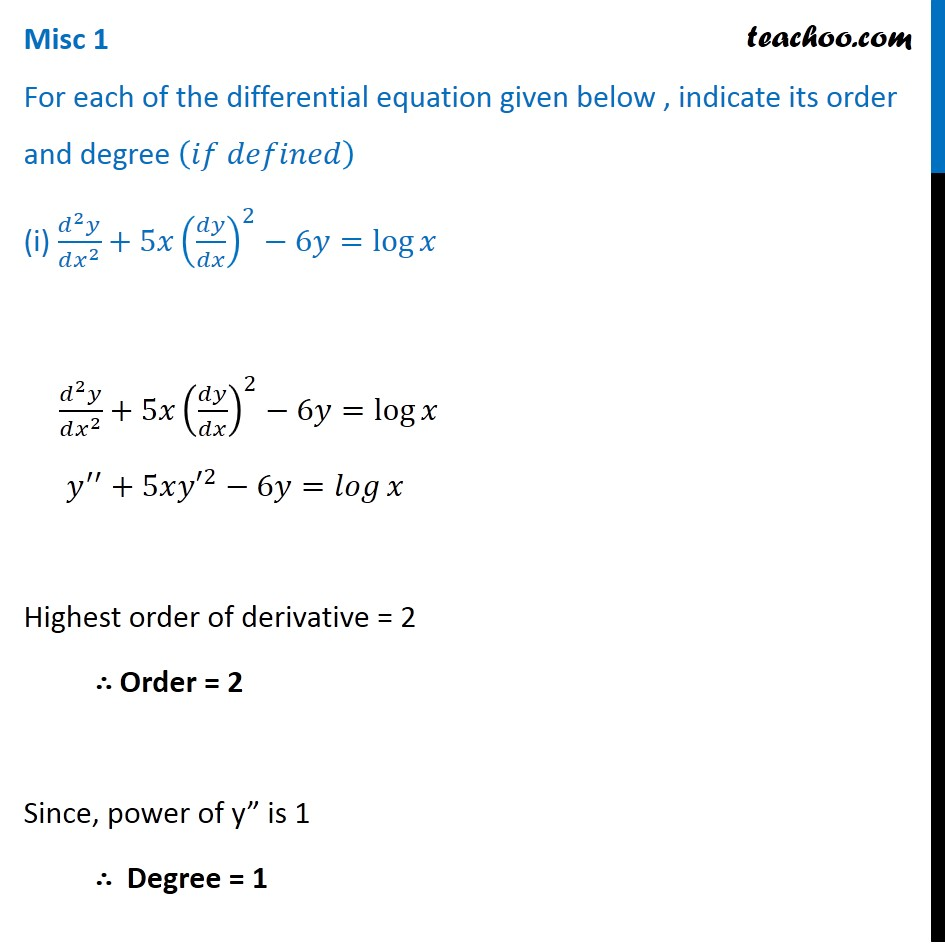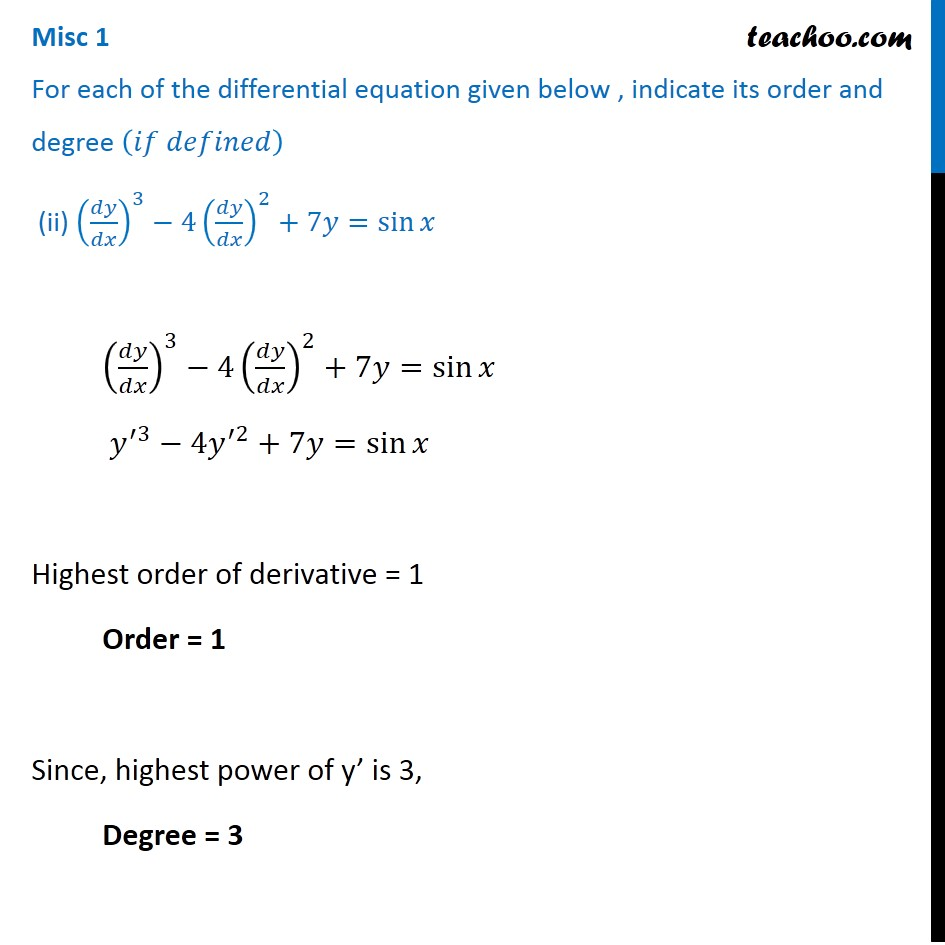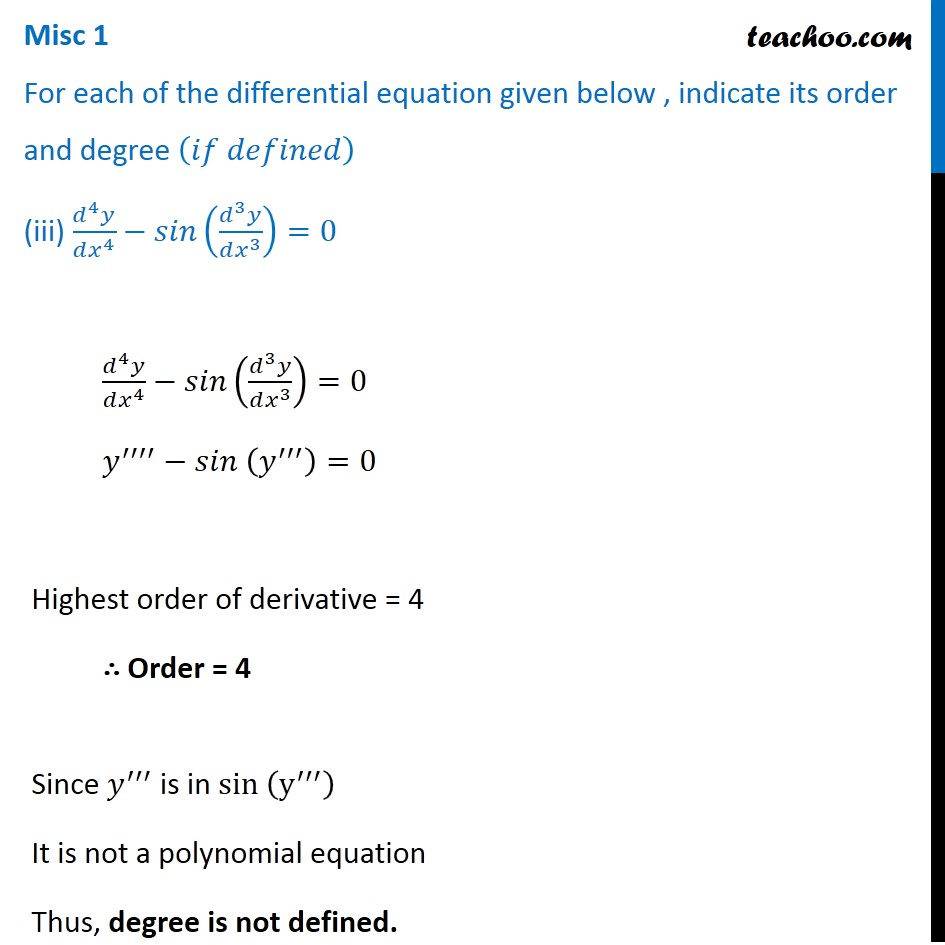Subscribe to our Youtube Channel - https://you.tube/teachoo

1. Chapter 9 Class 12 Differential Equations
2. Serial order wise
3. Miscellaneous

Transcript

Misc 1 For each of the differential equation given below , indicate its order and degree (𝑖𝑓 𝑑𝑒𝑓𝑖𝑛𝑒𝑑) (i) (𝑑^2 𝑦)/(𝑑𝑥^2 )+5𝑥(𝑑𝑦/𝑑𝑥)^2−6𝑦=log⁡𝑥 (𝑑^2 𝑦)/(𝑑𝑥^2 )+5𝑥(𝑑𝑦/𝑑𝑥)^2−6𝑦=log⁡𝑥 𝑦^′′+5𝑥〖𝑦′〗^2−6𝑦=𝑙𝑜𝑔⁡𝑥 Highest order of derivative = 2 ∴ Order = 2 Since, power of y” is 1 ∴ Degree = 1 Misc 1 For each of the differential equation given below , indicate its order and degree (𝑖𝑓 𝑑𝑒𝑓𝑖𝑛𝑒𝑑) (ii) (𝑑𝑦/𝑑𝑥)^3−4(𝑑𝑦/𝑑𝑥)^2+7𝑦=sin⁡𝑥 (𝑑𝑦/𝑑𝑥)^3−4(𝑑𝑦/𝑑𝑥)^2+7𝑦=sin⁡𝑥 𝑦^′3−4𝑦^′2+7𝑦=sin⁡𝑥 Highest order of derivative = 1 Order = 1 Since, highest power of y’ is 3, Degree = 3 Misc 1 For each of the differential equation given below , indicate its order and degree (𝑖𝑓 𝑑𝑒𝑓𝑖𝑛𝑒𝑑) (iii) (𝑑^4 𝑦)/(𝑑𝑥^4 )−𝑠𝑖𝑛((𝑑^3 𝑦)/(𝑑𝑥^3 ))=0 (𝑑^4 𝑦)/(𝑑𝑥^4 )−𝑠𝑖𝑛((𝑑^3 𝑦)/(𝑑𝑥^3 ))=0 𝑦^′′′′−𝑠𝑖𝑛 (𝑦^′′′ )=0 Highest order of derivative = 4 ∴ Order = 4 Since 𝑦^′′′ is in sin (y^′′′ ) It is not a polynomial equation Thus, degree is not defined.

Miscellaneous